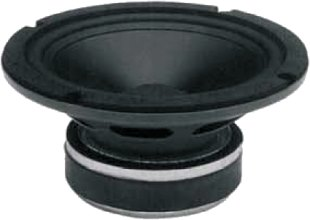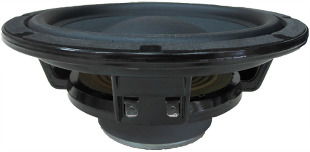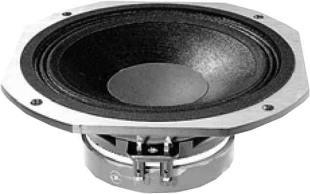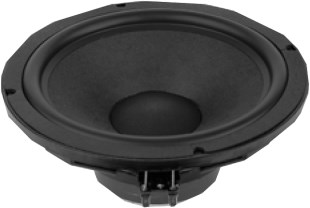# Beyma

Beyma is a well known producer of speaker in Sevilla, Spain.

## Tweeter## Beyma T 2030

order no. by-t2030

EUR 74,00excl. VAT: € 62.18 / \$ 67.16

25,4 mm dome tweeter with aluminium dome.

• power handling (continuous/programme) = 15/30 W
• frequency range = 1500-20000 Hz
• resonance frequency fs = 1050 Hz
• impedance R = 8 Ohm
• sound pressure level SPL = 95 dB (2,83V; 1m)
• DC resistance Re = 5 Ohm
• force factor BL = 3 N/A
• total Q factor Qts = 1,122 (Qms=3,957, Qes=1,567)
• voice coil diameter = 25,4 mm
• mounting diameter d = 84,5 mm
• overall diameter d = 102,5 mm
• mounting depth (not countersunk) t = 42 mm
• mass m = 0,7 kg
PDF data sheet

## Midsopen a bigger photo

recommended cabinet 1:
closed cabinet with 2,5 L volume
from 108/68 Hz (-3dB/-8dB)

recommended cabinet 2:
10,1 L volume bass reflex cabinet
with HP50 reflex tube, 14 cm long.
from 51/42 Hz (-3dB/-8dB).

## Beyma 5MP60/N

order no. by-5mp60n

EUR 79,30excl. VAT: € 66.64 / \$ 71.97

14 cm bass driver with paper cone.

• power handling (continuous/programme) = 50/100 W
• frequency range = 65-12000 Hz
• resonance frequency fs = 63 Hz
• impedance R = 8 Ohm
• sound pressure level SPL = 88 dB (2,83V; 1m)
• DC resistance Re = 5,3 Ohm
• force factor BL = 6,4 N/A
• voice coil inductance L = 0,2 mH
• effective piston radiating area Sd = 850 cm2
• effective mechanical mass incl. air load mms = 11 g
• equivalent volume of compliance Vas = 5,8 l
• total Q factor Qts = 0,46 (Qms=2,3, Qes=0,58)
• voice coil diameter = 25,4 mm
• maximum peak linear excursion vibration xlin = +/- 5,5 mm
• mounting diameter d = 120 mm
• overall diameter d = 150 mm
• mounting depth (not countersunk) t = 68 mm
• mass m = 1,2 kg
PDF data sheet## Beyma 5 M 30

order no. by-5m30

EUR 59,20excl. VAT: € 49.75 / \$ 53.73

12 cm midrange driver with paper cone.

• power handling (continuous/programme) = 50/100 W
• frequency range = 60-12000 Hz
• resonance frequency fs = 85 Hz
• impedance R = 8 Ohm
• sound pressure level SPL = 91 dB (2,83V; 1m)
• DC resistance Re = 6,5 Ohm
• force factor BL = 6,4 N/A
• voice coil inductance L = 0,5 mH
• effective piston radiating area Sd = 90 cm2
• effective mechanical mass incl. air load mms = 8 g
• equivalent volume of compliance Vas = 6 l
• total Q factor Qts = 0,61 (Qms=2,72, Qes=0,8)
• voice coil diameter = 25,8 mm
• maximum peak linear excursion vibration xlin = +/- 1 mm
• mounting diameter d = 120 mm
• overall diameter d = 134 mm
• mounting depth (not countersunk) t = 65 mm
• mass m = 1,09 kg
PDF data sheet## Beyma 6 B 30/P

order no. by-6b30p

EUR 57,20excl. VAT: € 48.07 / \$ 51.91

15 cm midrange driver with high quality cone.

• power handling (continuous/programme) = 50 W
• frequency range = 60-10000 Hz
• resonance frequency fs = 55 Hz
• impedance R = 8 Ohm
• sound pressure level SPL = 90 dB (2,83V; 1m)
• DC resistance Re = 5,9 Ohm
• voice coil inductance L = 0,5 mH
• effective piston radiating area Sd = 140 cm2
• equivalent volume of compliance Vas = 18 l
• total Q factor Qts = 0,62 (Qms=3,36, Qes=0,76)
• voice coil diameter = 26 mm
• maximum peak linear excursion vibration xlin = +/- 4 mm
• mounting diameter d = 140 mm
• overall diameter d = 166 mm
• mounting depth (not countersunk) t = 78 mm
• mass m = 1,25 kg
PDF data sheet

## 8 inch wooferopen a bigger photo

recommended cabinet 1:
closed cabinet with 33 L volume
from 55/35 Hz (-3dB/-8dB)

recommended cabinet 2:
134 L volume bass reflex cabinet
with HP100 reflex tube, 14 cm long.
from 26/22 Hz (-3dB/-8dB).

## Beyma 8 WOOFER/P V2

order no. by-8wooferpv2

EUR 89,40excl. VAT: € 75.13 / \$ 81.14

20 cm bass driver with high quality cone.

• power handling (continuous/programme) = 50 W
• frequency range = 30-6500 Hz
• resonance frequency fs = 35 Hz
• impedance R = 8 Ohm
• sound pressure level SPL = 90 dB (2,83V; 1m)
• DC resistance Re = 5,8 Ohm
• voice coil inductance L = 0,9 mH
• effective piston radiating area Sd = 220 cm2
• equivalent volume of compliance Vas = 59 l
• total Q factor Qts = 0,5 (Qms=2,72, Qes=0,62)
• voice coil diameter = 26 mm
• maximum peak linear excursion vibration xlin = +/- 4,5 mm
• mounting diameter d = 182,5 mm
• overall diameter d = 211,5 mm
• mounting depth (not countersunk) t = 76,5 mm
• mass m = 1,3 kg
PDF data sheet

## 10 inch wooferopen a bigger photo

recommended cabinet 1:
closed cabinet with 21 L volume
from 52/33 Hz (-3dB/-8dB)

recommended cabinet 2:
87 L volume bass reflex cabinet
with HP100 reflex tube, 30 cm long.
from 24/20 Hz (-3dB/-8dB).

## Beyma 10 BR 60 V2

order no. by-10br60v2

EUR 130,00excl. VAT: € 109.24 / \$ 117.98

25 cm bass driver with high quality cone.

• power handling (continuous/programme) = 100/200 W
• frequency range = 30-5000 Hz
• resonance frequency fs = 31 Hz
• impedance R = 8 Ohm
• sound pressure level SPL = 90,6 dB (2,83V; 1m)
• DC resistance Re = 6,5 Ohm
• voice coil inductance L = 1,5 mH
• effective piston radiating area Sd = 380 cm2
• equivalent volume of compliance Vas = 47 l
• total Q factor Qts = 0,47 (Qms=3,29, Qes=0,55)
• voice coil diameter = 52 mm
• maximum peak linear excursion vibration xlin = +/- 6,5 mm
• mounting diameter d = 232 mm
• overall diameter d = 260,5 mm
• mounting depth (not countersunk) t = 111 mm
• mass m = 2,87 kg
PDF data sheet

## Bass Shaker## Beyma SK07

order no. by-sk07

EUR 221,00excl. VAT: € 185.71 / \$ 200.57

.

• power handling (continuous/programme) = 50/100 W
• resonance frequency fs = 47 Hz
• impedance R = 16 Ohm
• voice coil diameter = 77 mm
• mass m = 1,5 kg
PDF data sheet

## no longer sold## Beyma HT3100

order no. not-available- not available

9 cm coax driver with paper cone.

• power handling (continuous/programme) = 6 W
• frequency range = 100-20000 Hz
• impedance R = 8 Ohm
• sound pressure level SPL = 85 dB (2,83V; 1m)
• mounting diameter d = 91 mm
• overall diameter d = 103,5 mm
• mounting depth (not countersunk) t = 85 mm
• mass m = 0,43 kg

## Beyma SE6VT

order no. not-available- not available

19 cm coax driver with paper cone.

• power handling (continuous/programme) = 6 W
• frequency range = 60-20000 Hz
• impedance R = 8 Ohm
• sound pressure level SPL = 90 dB (2,83V; 1m)
• mounting diameter d = 181 mm
• overall diameter d = 199 mm
• mounting depth (not countersunk) t = 104 mm
• mass m = 1 kg

## Beyma 8 AG/N

order no. not-available- not available

19 cm full range driver with paper cone.

• power handling (continuous/programme) = 35 W
• frequency range = 60-18000 Hz
• resonance frequency fs = 101 Hz
• impedance R = 8 Ohm
• sound pressure level SPL = 96 dB (2,83V; 1m)
• DC resistance Re = 7,5 Ohm
• force factor BL = 7,27 N/A
• voice coil inductance L = 0,32 mH
• effective piston radiating area Sd = 227 cm2
• effective mechanical mass incl. air load mms = 14 g
• equivalent volume of compliance Vas = 12,79 l
• total Q factor Qts = 0,98 (Qms=4,45, Qes=1,26)
• voice coil diameter = 35 mm
• mounting diameter d = 180 mm
• overall diameter d = 205 mm
• mounting depth (not countersunk) t = 84 mm
PDF data sheetopen a bigger photo

recommended cabinet 1:
closed cabinet with 54 L volume
from 46/29 Hz (-3dB/-8dB)

recommended cabinet 2:
212 L volume bass reflex cabinet
with HP100 reflex tube, 12 cm long.
from 21/18 Hz (-3dB/-8dB).

## Beyma 8 BR 40/N

order no. not-available- not available

20 cm midrange driver with high quality cone.

• power handling (continuous/programme) = 50 W
• frequency range = 35-6000 Hz
• resonance frequency fs = 33,2 Hz
• impedance R = 8 Ohm
• sound pressure level SPL = 90 dB (2,83V; 1m)
• DC resistance Re = 6,5 Ohm
• voice coil inductance L = 0,8 mH
• effective piston radiating area Sd = 220 cm2
• equivalent volume of compliance Vas = 61,35 l
• total Q factor Qts = 0,57 (Qms=2,61, Qes=0,73)
• voice coil diameter = 26 mm
• maximum peak linear excursion vibration xlin = +/- 6 mm
• mounting diameter d = 182,5 mm
• overall diameter d = 211,5 mm
• mounting depth (not countersunk) t = 77,5 mm
• mass m = 1,36 kg
PDF data sheet## Beyma 8 M 60 N

order no. not-available- not available

20 cm midrange driver with paper cone.

• power handling (continuous/programme) = 70/140 W
• frequency range = 120-9000 Hz
• resonance frequency fs = 87 Hz
• impedance R = 8 Ohm
• sound pressure level SPL = 95 dB (2,83V; 1m)
• DC resistance Re = 6,48 Ohm
• force factor BL = 9,1 N/A
• voice coil inductance L = 0,4 mH
• effective piston radiating area Sd = 210 cm2
• effective mechanical mass incl. air load mms = 18 g
• equivalent volume of compliance Vas = 11,3 l
• total Q factor Qts = 0,6 (Qms=2,679, Qes=0,778)
• voice coil diameter = 37,6 mm
• maximum peak linear excursion vibration xlin = +/- 1 mm
• mounting diameter d = 182 mm
• overall diameter d = 214 mm
• mounting depth (not countersunk) t = 86 mm
• mass m = 2,3 kg
PDF data sheet## Beyma MC 115

order no. not-available- not available

44,3 mm mids dome with high quality dome.

• power handling (continuous/programme) = 25 W
• frequency range = 600-12500 Hz
• impedance R = 8 Ohm
• sound pressure level SPL = 91 dB (2,83V; 1m)
• DC resistance Re = 6,13 Ohm
• force factor BL = 7,1 N/A
• total Q factor Qts = 1,127 (Qms=4,894, Qes=1,464)
• voice coil diameter = 44,3 mm
• mounting diameter d = 115 mm
• overall diameter d = 140 mm
• mounting depth (not countersunk) t = 40 mm
• mass m = 1,325 kg
PDF data sheet

## Beyma CL5T

order no. not-available- not available

20 cm coax driver with polypropylene cone.

• power handling (continuous/programme) = 30 W
• frequency range = 55-20000 Hz
• impedance R = 8 Ohm
• sound pressure level SPL = 89 dB (2,83V; 1m)
• mounting diameter d = 175 mm
• overall diameter d = 213 mm
• mounting depth (not countersunk) t = 147 mm
• mass m = 1,5 kg## Beyma IW5

order no. not-available- not available

coax driver with polypropylene cone.

• power handling (continuous/programme) = 40 W
• frequency range = 20-20000 Hz
• impedance R = 8 Ohm
• sound pressure level SPL = 87 dB (2,83V; 1m)
• mounting diameter d = 264x179 mm
• overall diameter d = 299x214 mm
• mounting depth (not countersunk) t = 115 mm
• mass m = 2 kg## Beyma IW6

order no. not-available- not available

coax driver with polypropylene cone.

• power handling (continuous/programme) = 50 W
• frequency range = 20-20000 Hz
• impedance R = 8 Ohm
• sound pressure level SPL = 88 dB (2,83V; 1m)
• mounting diameter d = 272x187 mm
• overall diameter d = 308x220 mm
• mounting depth (not countersunk) t = 127 mm
• mass m = 2,6 kgrecommended cabinet 1:
closed cabinet with 20 L volume
from 77/49 Hz (-3dB/-8dB)

recommended cabinet 2:
82 L volume bass reflex cabinet
with HP100 reflex tube, 11 cm long.
from 36/30 Hz (-3dB/-8dB).

## Beyma 10 BR 60

order no. not-available- not available

26 cm midrange driver with high quality cone.

• power handling (continuous/programme) = 100 W
• frequency range = 30-5000 Hz
• resonance frequency fs = 45 Hz
• impedance R = 8 Ohm
• sound pressure level SPL = 91,5 dB (2,83V; 1m)
• DC resistance Re = 5,6 Ohm
• voice coil inductance L = 1,1 mH
• effective piston radiating area Sd = 380 cm2
• equivalent volume of compliance Vas = 47 l
• total Q factor Qts = 0,47 (Qms=1,9, Qes=0,62)
• voice coil diameter = 52 mm
• maximum peak linear excursion vibration xlin = +/- 6 mm
• mounting diameter d = 240 mm
• overall diameter d = 270 mm
• mounting depth (not countersunk) t = 111 mm
PDF data sheetrecommended cabinet 1:
closed cabinet with 37 L volume
from 52/33 Hz (-3dB/-8dB)

recommended cabinet 2:
152 L volume bass reflex cabinet
with HP100 reflex tube, 14 cm long.
from 24/20 Hz (-3dB/-8dB).

## Beyma 8 BR 40

order no. not-available- not available

20 cm midrange driver with high quality cone.

• power handling (continuous/programme) = 50 W
• frequency range = 35-6000 Hz
• resonance frequency fs = 31 Hz
• impedance R = 8 Ohm
• sound pressure level SPL = 90 dB (2,83V; 1m)
• DC resistance Re = 6,3 Ohm
• voice coil inductance L = 0,7 mH
• effective piston radiating area Sd = 220 cm2
• equivalent volume of compliance Vas = 82 l
• total Q factor Qts = 0,48 (Qms=2,29, Qes=0,6)
• voice coil diameter = 26 mm
• maximum peak linear excursion vibration xlin = +/- 6 mm
• mounting diameter d = 182 mm
• overall diameter d = 216 mm
• mounting depth (not countersunk) t = 83 mm
PDF data sheetrecommended cabinet 1:
closed cabinet with 19 L volume
from 72/45 Hz (-3dB/-8dB)

recommended cabinet 2:
71 L volume bass reflex cabinet
with HP100 reflex tube, 17 cm long.
from 36/29 Hz (-3dB/-8dB).

## Beyma 8 WOOFER/P

order no. not-available- not available

20 cm bass driver with paper cone.

• power handling (continuous/programme) = 50 W
• frequency range = 30-3500 Hz
• resonance frequency fs = 35 Hz
• impedance R = 8 Ohm
• sound pressure level SPL = 92 dB (2,83V; 1m)
• DC resistance Re = 5,37 Ohm
• voice coil inductance L = 0,6 mH
• effective piston radiating area Sd = 220 cm2
• equivalent volume of compliance Vas = 75 l
• total Q factor Qts = 0,39 (Qms=2,33, Qes=0,47)
• voice coil diameter = 26 mm
• maximum peak linear excursion vibration xlin = +/- 4,5 mm
• mounting diameter d = 182 mm
• overall diameter d = 214 mm
• mounting depth (not countersunk) t = 86 mm
PDF data sheet

## Beyma 8 BX Koaxial

order no. not-available- not available

20 cm coax driver with polypropylene cone.

• power handling (continuous/programme) = 100/200 W
• frequency range = 25-20000 Hz
• resonance frequency fs = 52 Hz
• impedance R = 8 Ohm
• sound pressure level SPL = 92 dB (2,83V; 1m)
• DC resistance Re = 5,5 Ohm
• force factor BL = 7,3 N/A
• voice coil inductance L = 0,2 mH
• effective piston radiating area Sd = 220 cm2
• effective mechanical mass incl. air load mms = 23 g
• equivalent volume of compliance Vas = 23 l
• total Q factor Qts = 0,68 (Qms=6,1, Qes=0,77)
• voice coil diameter = 38,5 mm
• maximum peak linear excursion vibration xlin = +/- 5 mm
• mounting diameter d = 182 mm
• overall diameter d = 216 mm
• mounting depth (not countersunk) t = 120 mm
• mass m = 2,9 kg
PDF data sheet## Beyma T 2010

order no. not-available- not available

25,8 mm dome tweeter with high quality dome.

• power handling (continuous/programme) = 12/24 W
• frequency range = 1500-20000 Hz
• impedance R = 8 Ohm
• sound pressure level SPL = 92 dB (2,83V; 1m)
• DC resistance Re = 6 Ohm
• force factor BL = 3,2 N/A
• total Q factor Qts = 1,638 (Qms=5,185, Qes=2,394)
• voice coil diameter = 25,8 mm
• mounting diameter d = 84,5 mm
• overall diameter d = 100 mm
• mounting depth (not countersunk) t = 29 mm
• mass m = 0,6 kg
PDF data sheet

## Beyma CL6T

order no. not-available- not available

22 cm coax driver with polypropylene cone.

• power handling (continuous/programme) = 45 W
• frequency range = 45-20000 Hz
• impedance R = 8 Ohm
• sound pressure level SPL = 89 dB (2,83V; 1m)
• mounting diameter d = 205 mm
• overall diameter d = 239 mm
• mounting depth (not countersunk) t = 172 mm
• mass m = 2 kg
PDF data sheet

## Beyma CL8T

order no. not-available- not available

26 cm coax driver with polypropylene cone.

• power handling (continuous/programme) = 55 W
• frequency range = 40-20000 Hz
• impedance R = 8 Ohm
• sound pressure level SPL = 91 dB (2,83V; 1m)
• mounting diameter d = 245 mm
• overall diameter d = 280 mm
• mounting depth (not countersunk) t = 210 mm
• mass m = 2,8 kg
PDF data sheetrecommended cabinet 1:
closed cabinet with 32 L volume
from 78/49 Hz (-3dB/-8dB)

recommended cabinet 2:
112 L volume bass reflex cabinet
with 2x HP100 reflex tube, 15 cm long.
from 40/32 Hz (-3dB/-8dB).

## Beyma 12 BR 70

order no. not-available- not available

30 cm bass driver with high quality cone.

• power handling (continuous/programme) = 125 W
• frequency range = 25-2500 Hz
• resonance frequency fs = 35 Hz
• impedance R = 8 Ohm
• sound pressure level SPL = 94,5 dB (2,83V; 1m)
• DC resistance Re = 5,6 Ohm
• voice coil inductance L = 1 mH
• effective piston radiating area Sd = 540 cm2
• equivalent volume of compliance Vas = 154 l
• total Q factor Qts = 0,36 (Qms=1,85, Qes=0,44)
• voice coil diameter = 52 mm
• maximum peak linear excursion vibration xlin = +/- 6 mm
• mounting diameter d = 286 mm
• overall diameter d = 320 mm
• mounting depth (not countersunk) t = 125 mm
PDF data sheetrecommended cabinet 1:
closed cabinet with 21 L volume
from 77/49 Hz (-3dB/-8dB)

recommended cabinet 2:
62 L volume bass reflex cabinet
with 2x HP100 reflex tube, 36 cm long.
from 43/33 Hz (-3dB/-8dB).

## Beyma 12 B 100/R

order no. not-available- not available

30 cm bass driver with high quality cone.

• power handling (continuous/programme) = 150 W
• frequency range = 25-2500 Hz
• resonance frequency fs = 29 Hz
• impedance R = 8 Ohm
• sound pressure level SPL = 93,5 dB (2,83V; 1m)
• DC resistance Re = 6,6 Ohm
• voice coil inductance L = 1,3 mH
• effective piston radiating area Sd = 530 cm2
• equivalent volume of compliance Vas = 154 l
• total Q factor Qts = 0,3 (Qms=6,49, Qes=0,31)
• voice coil diameter = 100 mm
• maximum peak linear excursion vibration xlin = +/- 3 mm
• mounting diameter d = 286 mm
• overall diameter d = 320 mm
• mounting depth (not countersunk) t = 125 mm
PDF data sheetopen a bigger photo

recommended cabinet 1:
closed cabinet with 23 L volume
from 95/60 Hz (-3dB/-8dB)

recommended cabinet 2:
56 L volume bass reflex cabinet
with 2x HP100 reflex tube, 24 cm long.
from 57/43 Hz (-3dB/-8dB).

## Beyma 15 B 100R/GH

order no. not-available- not available

37 cm bass driver with high quality cone.

• power handling (continuous/programme) = 400/800 W
• frequency range = 40-4500 Hz
• resonance frequency fs = 30 Hz
• impedance R = 8 Ohm
• sound pressure level SPL = 98 dB (2,83V; 1m)
• DC resistance Re = 5,4 Ohm
• force factor BL = 21,8 N/A
• voice coil inductance L = 1,6 mH
• effective piston radiating area Sd = 880 cm2
• effective mechanical mass incl. air load mms = 119 g
• equivalent volume of compliance Vas = 249 l
• total Q factor Qts = 0,25 (Qms=10,894, Qes=0,258)
• voice coil diameter = 100 mm
• maximum peak linear excursion vibration xlin = +/- 6 mm
• mounting diameter d = 355 mm
• overall diameter d = 388 mm
• mounting depth (not countersunk) t = 145 mm
PDF data sheet

The cabinet volume calculations are executed considering an external resistance of 0.3 Ohm. We are using, if no other data is known or available, the information supplied by the manufacturer.

Please note: the frequency response doesn't say much about the cut-off frequency inside the cabinet! Therefore, we mostly give you 2 examples with dimensioning considerations and an indication, how low the loudspeaker will reproduce.

Home